## Saturday, 9 August 2014

### chapter 3 Concept of Infinity

• Infinity does not stand for any particular real number. In fact, it cannot be defined precisely. This is a deep concept. For any number you can think of, no matter how large, infinity is still larger. When we say that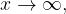$x \to \infty ,$ we mean that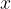$x$ increase in an unbounded fashion, that is, becomes indefinitely large.
• We cannot apply the normal rules of arithmetic to infinity. For example, saying that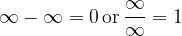$\infty - \infty = 0\,{\rm{or}}\,\dfrac{\infty }{\infty } = 1$
is absurd because such quantities are not defined.
• It should be clear now that an expression like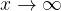$x \to \infty$ defines a tendency (of unbounded increase)
Consider a dfraction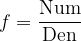$f = \dfrac{{{\rm{Num}}}}{{{\rm{Den}}}}$
As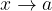$x \to a$, if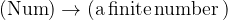$({\rm{Num}}) \to ({\rm{a \,finite \,number\,}})$ and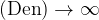$({\rm{Den}}) \to \infty$ , then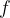$f$tends to become infinitesmally small or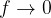$f \to 0$.
Such cases are listed out below. Observe the details carefully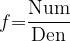$f{\rm{ = }}\dfrac{{{\rm{Num}}}}{{{\rm{Den}}}}$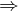$\Rightarrow$ Suppose that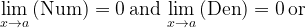$\mathop {\lim }\limits_{x \to a} \left( {{\rm{Num}}} \right) = 0\,{\rm{and}}\,\mathop {\lim }\limits_{x \to a} \left( {{\rm{Den}}} \right) = 0\,{\rm{or}}\,$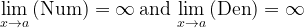$\mathop {\lim }\limits_{x \to a} \left( {{\rm{Num}}} \right) = \infty \,{\rm{and}}\,\mathop {\lim }\limits_{x \to a} \left( {{\rm{Den}}} \right) = \infty$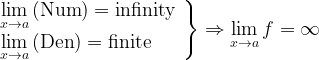$\left. \begin{array}{l} \mathop {{\rm{lim}}}\limits_{x \to a} \left( {{\rm{Num}}} \right) = {\rm{infinity}}\\ \mathop {{\rm{lim}}}\limits_{x \to a} \left( {{\rm{Den}}} \right)= {\rm{finite}} \end{array} \right\} \Rightarrow \mathop {{\rm{lim}}}\limits_{x \to a} f = \infty$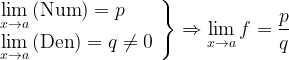$\left. \begin{array}{l} \mathop {{\rm{lim}}}\limits_{x \to a} \left( {{\rm{Num}}} \right) = p\\ \mathop {{\rm{lim}}}\limits_{x \to a} \left( {{\rm{Den}}} \right) = q \ne 0 \end{array} \right\} \Rightarrow \mathop {{\rm{lim}}}\limits_{x \to a} f = \dfrac{p}{q}$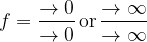$f = \dfrac{{ \to 0}}{{ \to 0}}\,{\rm{or}}\,\dfrac{{ \to \infty }}{{ \to \infty }}$
Then$f$ is of the form:
The tendency (or limits) of such forms may or may not exist
For example, if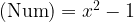$({\rm{Num}}) = {x^2} - 1$ and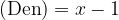$({\rm{Den}}) = x - 1$, then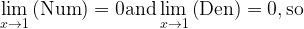$\mathop {\lim }\limits_{x \to 1} \left( {{\rm{Num}}} \right) = 0 {\rm{and}}\mathop {\lim }\limits_{x \to 1} \left( {{\rm{Den}}} \right) = 0,{\rm{so}}$ that$f$ is of the form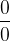$\dfrac{0}{0}$. But as we have seen earlier, the limit of$f$ exists, as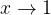$x \to 1$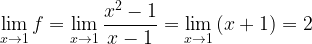$\mathop {\lim }\limits_{x \to 1} f = \mathop {\lim }\limits_{x \to 1} \dfrac{{{x^2} - 1}}{{x - 1}} = \mathop {\lim }\limits_{x \to 1} \left( {x + 1} \right) = 2$
Limits of the form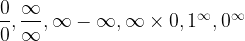$\dfrac{0}{0},\dfrac{\infty }{\infty },\infty - \infty ,\infty \times 0,{1^\infty },{0^\infty }$ are called indeterminate forms. The limits of such forms may exist but it cannot be determined by simple observation (hence the name indeterminate). Such forms need to be reduced into determinate forms for which the limit can be determined. In the above case, for example, an indeterminate form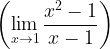$\left( {\mathop {\lim }\limits_{x \to 1} \dfrac{{{x^2} - 1}}{{x - 1}}} \right)$ was reduced into a determinate form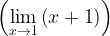$\left( {\mathop {\lim }\limits_{x \to 1} \left( {x + 1} \right)} \right)$, whose limit can be determined by substitution of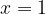$x = 1$